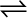7.30 At 473 K, equilibrium constant Kc for decomposition of phosphorus pentachloride, PCl5 is 8.3 ×10-3. If decomposition is depicted as,

PCl5 (g)PCl3 (g) + Cl2 (g)  ∆r = 124.0 kJ mol–1

a) write an expression for Kc for the reaction.

b) what is the value of Kc for the reverse reaction at the same temperature?

c) what would be the effect on Kc if (i) more PCl5 is added (ii) pressure is increased (iii) the temperature is increased?

(b) Value of Kc for the reverse reaction at the same temperature is:

(c)

(i) ${\mathrm{K}}_{\mathrm{c}}$ would remain the same because in this case, the temperature remains the same.

(ii) ${\mathrm{K}}_{\mathrm{c}}$ is constant at a constant temperature. Thus, in this case, ${\mathrm{K}}_{\mathrm{c}}$ would not change.

(iii)In an endothermic reaction, the value of ${\mathrm{K}}_{\mathrm{c}}$ increases with an increase in temperature. Since the given reaction in an endothermic reaction, the value of ${\mathrm{K}}_{\mathrm{c}}$ will increase if the temperature is increased.